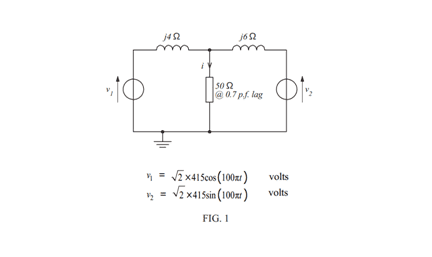# Norton Generators Question

• Engineering
Joe85
Homework Statement:
Hi all,

I realise that this question has been asked numerous times in relation to Parts A and B but am strugging to find any ground covered on Part C. I believe i have answered this correctly but the wording of the question leads me to believe perhaps i have missinterpreted what was being asked.

The Question:

FIGURE 1 shows a 50 Ω load being fed from two voltage sources via
their associated reactances. Determine the current i flowing in the load by:

(a) applying Thévenin’s theorem
(b) applying the superposition theorem
(c) by transforming the two voltage sources and their associated
reactances into current sources (and thus form a pair of Norton
generators)

Relevant Equations:
.V1 = 415cos(100πt)
V2 = 415sin(100πt)
Load Impedance = 50Ω (0.7 Pf Lag) = 35.002 + J35.705Ω
Converting V1 & V2 to RMS values and V1 to a sin value:
V1 = 415cos(100πt)
V2 = 415cos(100πt-90)
and from Asin(ωt+Φ)
V1 = 415∠0V or 415 +J0V
V2 = 415∠-90V or 0-j415 V

Intially, i convert the the volage sources into current sources:

I1 = V1/J4 = -J103.75A
I2 = V2/J6 = -69.16666667A

I then red-draw the circuit with the voltage sources replaced with current sources and the series impedances placed in parallel with the current source.

Next step was to remove the load impedance and replace with a short circuit. I then took the current through the Short Circuit (Points A & B):

Isc = I1+I2 = -69.16666667 - J103.75A.

Next step was to replace the the current sources with Open Circuits and remove the short circuit that was placed beween Points A & B.

With the Two Reactances in parallel:

Zn = (Z1Z2)/(Z1+Z2)
Zn = J2.4Ω

Circuit is now redrawn as a Norton Equvalent with Isc in parallel with Zn and an open circuit between Points A & B.

Once again, redrew the circuit again placing the Load (ZL) back into the circuit between points A & B.

Zn & Zl now in parallel - Product over sum rule to reduce to a total impedance Zt:

Zt = (ZnZL)/(Zn+ZL) = 0.07530879804 + J2.318014921Ω

Calculated the Load Voltage using Ohms Law:

VL = Isc × Zt = 235.2851896 - J168.1426532.

Finally, i calculated the load current utilising Ohms Law on Load impedance:

IL = VL/ZL = 0.8927 -J5.714A.

The answer is identical to my answer for parts A & B (to 4 sf - may revist the calculations to tidy up), just not sure the method I've used is what they were asking.

Thanks,

Joe.

•